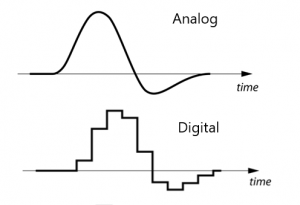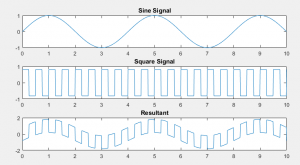# Basic Signal processing

### What is Basic Signal Processing?

Signal Processing refers to any kind of modification or analysis of a signal. These processing techniques are used to improve the efficiency of the system. Signal processing has applications in nearly every field of life, but before we get into that, let us define signal.

A signal is an electrical impulse or a wave that carries information. This refers to the changing currents, or voltages, or electromagnetic waves that transmit data at any point in electrical systems. Let us briefly go through its types

### Basic Signal Processing type of signals

Basically, there are two types of signals

• Analog: Analog signal is a continuous time-varying signal that has an infinite amount of samples in it. These analog signals are further divided into composite and simple analog signals. Sinusoidal waves being simple analog signals and all the others, composite signals, are made from the combination of multiple variants of simple sinusoidal waves.
• Digital : Digital signals also carry data just like analog signals, but in the case of digital signals, these values are discrete. It represents data in the form of a discrete sequence of values and is defined by interval or the bit rate. The digital signals thus contain a set of numbers that depict the samples of a continuous variable.

###The picture above represents the same signal in both analog and digital form. Note that the edges in the digital signal represent the point at which the sample was taken.

Now let us move towards some basic signal processing techniques.

### Basic Operations in Signal Processing

• Addition: The addition in signal is quite similar to the traditional mathematical addition. In order to add two signals together, we add the amplitude of both present at the same timestamp. Mathematically, if one signal is X1(t) and the other is X2(t), then the result will be denoted by

Output = X1(t) + X2(t)

###The figure below demonstrates the addition of the two signals

• Subtraction : The subtraction of two signals is similar to addition. We just subtract the amplitude of the second signal from the first one. Mathematically, if one signal is X1(t) and the other is X2(t), then the resultant will be denoted by

Output = X1(t) – X2(t)The image above shows the subtraction of the same signals used in the addition example. Note that the pattern looks quite similar, but the edges are different. This is because the amplitude, in this case, is subtracted.

• Sampling : Sampling is the most fundamental technique used in signal processing. It is used to reduce the sample size of a given signal, and you can also change an analog signal to a digital signal using sampling. In sampling, we measure the instantaneous value of the input signal after each interval. This interval also determines the sampling frequency of the system.

For example, if we consider the previous signal, we can sample it by taking values at a certain point in time. This will convert the signal into a discrete form also known as digital form as shown in the pictureNote that the dotted line in the sampled signal there only represents the original wave.

• Time Shifting: Time-shifting refers to the movement of the signal along the horizontal axis. Time-shifting does not change a signal’s attribute; it just changes its origin. Let us consider the following signal,If you want to shift this signal by three, then it will becomeThe signal above is shifted towards the right, which means that the signal is delayed. If the time shift is negative, then the signal will shift towards the left, i.e., the signal will advance. Note that the amplitude and the dimensions of the signal are the same; only the origin has changed in time-shifting.

• Time Scaling: The compression and expansion of a signal along the horizontal axis is known as time-scaling. This can be done by multiplying the time component of the signal with a constant number. If the number is greater than 1 then the signal will compress. If the number is less than 1, then the signal will expand. The figure below shows the compression and expansion of the signals.Note that the amplitude of the signal is the same along with the axis. The signal will get slow as it gets wider and will become fast when it is compressed. Slow and fast refers to the frequency of the signal, i.e., slow signals have low frequency, and fast signals have a high frequency.

• Time Inversion : If we flip the signal on the y-axis, it becomes inverted; this process is known to be time inversion of a signal. The inverted signal is the mirror image of the original signal, and there is no change in the amplitude or the dimensions of the image. The image below shows the original and the inverted sine signalTime inversion can be considered as a special case of time scaling in which we scale the signal by -1. Remember that time inversion and time reversal are different operations. In time-reversal, the signal is replicated in the opposite direction on the x-axis.

All these time-domain operations can be performed on the frequency domain and amplitude as well. For example, amplitude scaling will work the same way except that it will only affect the amplitude axis and not the time.

• Multiplication : Multiplication of the signal is similar to the addition technique. In multiplication, the parent signal is multiplied with the amplitude of the other signal at every instant. If parent signal is X1(t) and the other is X2(t), then the resultant will be denoted by

Output = X1(t) X2(t)The figure shows the multiplication of two signals that were used in the previous example. The resultant has the same amplitude because the waves had a magnitude of 1. If the waves have amplitude > 1, then you can observe the change in amplitude as well. The figure below shows the change in amplitude.Note that the amplitude of the resultant wave has changed because the square wave has a magnitude of 4.